Courses

# Variational Principle : Euler-Lagrange Equation and its Applications (Part -1) - Classical Mechanics Physics Notes | EduRev

## Physics for IIT JAM, UGC - NET, CSIR NET

Created by: Akhilesh Thakur

## Physics : Variational Principle : Euler-Lagrange Equation and its Applications (Part -1) - Classical Mechanics Physics Notes | EduRev

The document Variational Principle : Euler-Lagrange Equation and its Applications (Part -1) - Classical Mechanics Physics Notes | EduRev is a part of the Physics Course Physics for IIT JAM, UGC - NET, CSIR NET.
All you need of Physics at this link: Physics

Introduction

We are familiar with Newton's equation of motion for a particle. A particle is an idealized body having physical attributes, such as, mass, charge etc. but whose dimensions can be neglected in describing its motion, i.e. it is considered to be a geometrical point.
Consider a single particle of mass m, whose position at time t is given by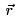(if we are using the Cartesian coordinates, we specify the position by x; y and z). Since the equation of motion is second order in time, if the positionand the velocity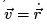of the particle is known at some initial time t = 0, we can in principle, by integrating the equation of motion, determine the position and the velocity for all time to come. This can become quite complicated if we consider complex systems, for instance, a system of N particles.
Since the position of each particle requires three quantities (such as x, y, z or r; θ, φ), in order to specify the positions of all the N particles simultaneously, we need to specify 3N quantities. However, it is likely that the system is under constraints. For instance, if we have two independent particles, we would require 6 coordinates, three for each. However, if the two particles are connected by a rigid rod, the distance between them remains xed as the two particles move. In such a case we need only ve quantities to specify the state of motion of the two particle system. In general, if the system of N particles have k independent constraints, the number of independent quantities would be 3N k. This is known as the degree of freedom of the system.The position of a system with d degrees of freedom at any time t is completely de ned by d generalized coordinates q1, q2, ......., qd.
In order to describe the mechanical state of the system, it is not enough to specify the generalized coordinates alone at time t = 0 because from a knowledge of these alone one cannot determine the position of the system at a subsequent time. In addition, we need to specify the velocities of each of the particles at this time as well. Since velocity is a vector, we need d number of generalized velocities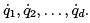. [Note: Integrating the equations of motion for such a system gets very complicated . what we need is alternative formulation of the equation of motion. This is provided by Lagrange's equations. The advantage of this \Analytical Mechanics" over the conventional vector mechanics are threefold. Firstly, it provides a single unifying principle to work with.
Second, unlike vector mechanics where each particle comprising a system has to be treated separately, the analytical mechanics looks at the system as a whole. Finally, while in vector mechanics the constraints are treated by invoking special forces., here they are taken care of in a natural way.

The Variational Principle

Before we discuss the classical variational principle, we would make a short but interesting digression. This has to do with Feynman's formulation of quantum mechanics using his path integral method.
We know from our knowledge of elementary quantum mechanics that the state of a particle at a position x at time t is given by the wave function ψ (x, t) which has the interpretation of probability amplitude of the particle to be at that position at time t. (Our discussion can be readily extended to three dimensions). Feynman had provided an interpretation of this amplitude in his Ph.D. thesis entitled "The Principle of Least action in quantum Mechanics", submitted to the Princeton University in 1942. His supervisor Wheeler was so much impressed with this novel interpretation of quantum mechanics that he is said to have remarked to Einstein "Does not this marvellous discovery make you willing to accept quantum mechanics?" (Einstein, who was always skeptical about quantum mechanics had retorted "I still cannot believe that God plays dice, but may be, I have earned the right to make my mistakes").
The idea behind the path integral formulation is as follows: Suppose a particle is at the position xat time ti. The quantum mechanical amplitude for the particle to be found at the position xat time tis given by summing over al l possible paths that connect xi to xf , i.e.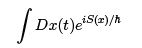where the weight factor for each path is determined by the classical action S (x, t).

The classical equation of motion is determined in a very simple way by taking the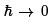limit. In this limit the weight factor oscillates very rapidly so that the contribution to the integral comes primarily from those paths for which the action is nearly stationary.
We will see later that this is just the way the EulerLagrange equations are derived from the classical action.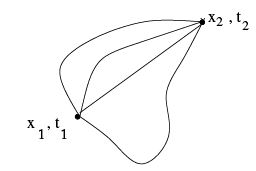Fig.1: Possible paths connecting two points

Let us look at a couple of examples before discussing the variational principle. First, let us consider the shortest distance between two points 1 and 2 on a plane. How does one nd this, which we intuitively know to be the straight-line joining the two points?
We could join the points 1 and 2 by drawing all possible curves, measure the length of each of the paths and nd which of them is of minimum length. The length of the curve is given by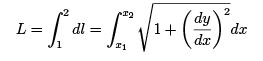(1)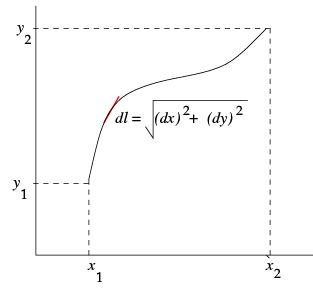Figure 2: Geometry of a typical path

We have to determine the function y(x) which minimises the length. L[y(x)] depends on the function y(x) itself. This is known as a functional. The way we have written seems to suggest that L[y(x)] is nothing but function of a function. However, there a subtle di
erence between a functional and function of a function. A function is a one to one mapping from a range of values in its domain to a value in its image plane or the range.
A functional takes as its argument not one particular value but a function itself and maps it to a de nite value. For different functions as its argument, the functional returns a different value.
Consider a second example. This is Fermat's principle of least time, which states that in travelling between two points, light would take that path for which the travel time is minimum. If the refractive index of the medium n(x) is constant, the shortest time is also the shortest path. However, if n(x) depends on x, the time to travel a distance dl is dt = dl/v = dl/(c/n) = ndl/c. Thus the total time taken in travelling from the point 1 to the point 2 is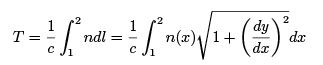(2)

In both these cases, we need to minimise the integral over di
erent possible paths. There could be situation where we may need to maximise or nd a point of in
ection. We will determine a general condition for such optimisation.
Recall that for the function of a single variable x, the maximum or the minimum is given by the vanishing of the rst derivative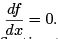If, however, the second derivative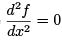as well, the function has a point of inction there.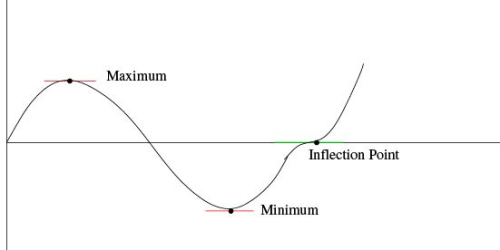Figure 3: Showing the stationary points

If we consider a function several variables F (x) where x = (x1, x2,......... xn), using a Taylor expansion, we have,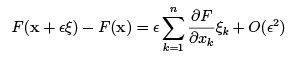We de ne a stationary point (maximum, minimum or a point of inection) as a point at which the rst order term in ε vanishes for all ξ , i.e.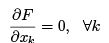We extend the same idea for a functional. Consider a functional F [y(x)]. How does the functional change with the change in the argument, which is a function y(x)? Suppose a particular path is given by y(x). The neighboring path can be written as y(x) + εh(x) where h(x) is some di
erentiable function. Suppose the end points of all the paths are (x1, y1) and (x2, y2). Since these two points are common to all the possible paths, we must have, for all the paths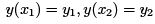which implies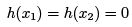Consider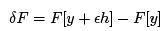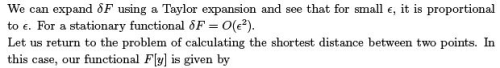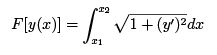so that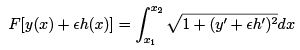Using a Taylor like expansion, we have,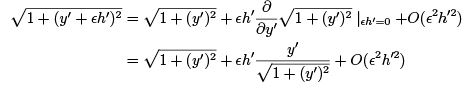Thus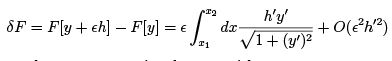As F is stationary, the term proportional to ε vanishes,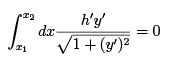It can be shown that a necessary and sucient condition for the above to be valid is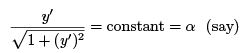The suffciency is readily proved for then we have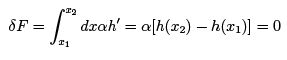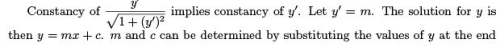Derivation of Euler-Lagrange Equation

We will derive a general condition for optimization of a functional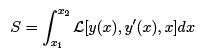(3)

where y(x) is a curve joining xand x2. The problem is to nd the path y(x) = y*(x) which makes S stationary.
Consider one parameter family of functions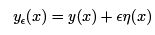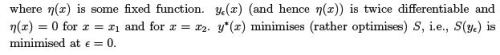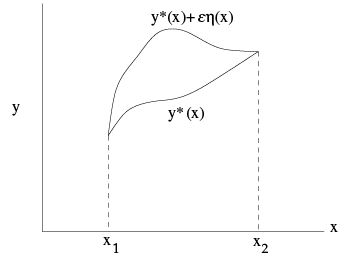Fig. 4: The optimal path at ε = 0 and another possible path

Now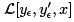is a function of a single variable ε. which must be satis ed at ε = 0. Since the integral is over x, the differentiation with respect to ε can be carried out inside the integral. So the condition for optimization is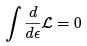Note that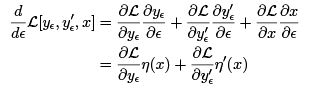Thus the condition of optimization is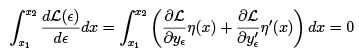The second term of the above can be integrated by parts to give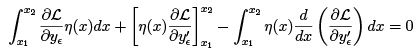The second term on the left is zero because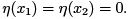We then have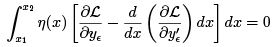(4)

as the condition for optimisation of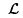. This equation holds for an arbitrary continuous function η(x). We claim that if we have an equation of the type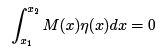for an arbitrary, continuous function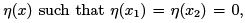, it follows that M (x) = 0 on [x1; x2].
Since the condition is valid for an arbitrary continuous function, consider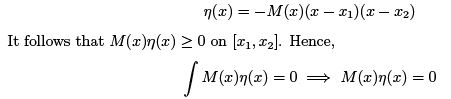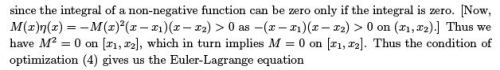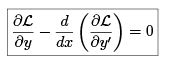Applications

Newton's Law from the Euler-Lagrange Equation

Consider a single particle in a potential V (x). The "action "integral is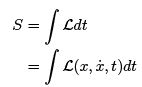(6)is known as the Lagrangian. Stationary action implies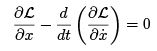If we define the Lagrangian as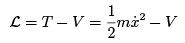(7)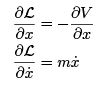Thus we get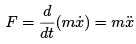which is just the Newton's law.

159 docs

,

,

,

,

,

,

,

,

,

,

,

,

,

,

,

,

,

,

,

,

,

;